# MA.912.AR.2.2Export Print
Write a linear two-variable equation to represent the relationship between two quantities from a graph, a written description or a table of values within a mathematical or real-world context.

### Clarifications

Clarification 1: Instruction includes the use of standard form, slope-intercept form and point-slope form, and the conversion between these forms.
General Information
Subject Area: Mathematics (B.E.S.T.)
Strand: Algebraic Reasoning
Status: State Board Approved

## Benchmark Instructional Guide

### Terms from the K-12 Glossary

• Linear Equation
• Slope
• $y$-intercept

### Vertical Alignment

Previous Benchmarks

Next Benchmarks

### Purpose and Instructional Strategies

In grade 8, students wrote linear two-variable equations in slope-intercept form from tables, graphs and written descriptions. In Algebra I, students write linear two-variable equations in all forms from real-world and mathematical contexts. In future courses, students will write systems and solve problems involving systems in three-variables and linear programming. Additionally, linear equations and linear functions are fundamental parts of all future high school courses.
• Instruction includes making connections to various forms of linear equations to show their equivalency. Students should understand and interpret when one form might be more useful than other depending on the context.
• Standard Form
Can be described by the equation $A$$x$ + $B$$y$ = C, where $A$, $B$ and $C$ are any rational number. This form can be useful when identifying the $x$- and $y$-intercepts.
• Slope-Intercept Form
Can be described by the equation $y$ = $m$$x$ + $b$, where $m$ is the slope and $b$ is the $y$-intercept. This form can be useful when identifying the slope and $y$-intercept.
• Point-Slope Form
Can be described by the equation $y$ − $y$1 = $m$($x$$x$1), where ($x$1, $y$1) are a point on the line and $m$ is the slope of the line. This form can be useful when a point on the line is given and the $y$-intercept is not easily determinable.
• Look for opportunities to point out the connection between linear contexts and constant rates of change.
• Problem types should include cases for vertical and horizontal lines.

### Common Misconceptions or Errors

• Students may have difficulty identifying both variables from a context. Much of their work previously has involved univariate contexts. Place emphasis on asking students what is changing in each context. Help guide their thoughts to recognize bivariate contexts as having two “things” that change in tandem.
• Students may attempt to estimate intercepts in order to continue using a linear form they prefer for some contexts. Use these opportunities to address the need for precision in mathematics.

### Strategies to Support Tiered Instruction

• Instruction includes strategies from MA.912.AR.1.2 on rearranging equations to help students when they convert from one form of two-variable linear equation to another.
• Teacher models opportunities to address the need for precision in mathematics when determining intercepts.
• For example, a student could prefer to use slope-intercept form when writing two variable linear equations. If the given information, as shown below, is two points that do not include the y-intercept, then the student may only estimate the $y$-intercept rather than determining it exactly. The student should realize that they could use point-slope form to write the equation without having to determine the $y$-intercept.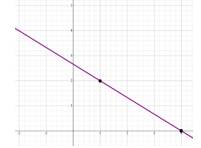• Instruction includes explicit questions such as “What is staying the same or constant?”, “What is changing or varying?” or “Is there anything else varying?”
• For example, students are given the situation where a dog groomer charges \$25 for a shampoo and hair cut plus \$10 for each hour the dog stays at the groomer and are asked to write a linear two-variable equation that represents the total cost. The teacher can provide questions to help determine the constant value and two variables.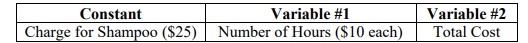• Instruction includes discussions about what a particular coordinate point on a line means in the context of the problem. Teachers may ask, “What does the identified point represent in the context of the problem?” and “How does the $y$ change as $x$ increases/decreases?”
• Teacher models finding the slope by color coding the points.
• For example, to find the slope of a line passing through points (1 2) and (4 0), you would use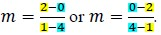• For students who need extra support in adding or subtracting integers, instruction includes using two-colored counters or algebra tiles to model the operation.
• For example, given the expression 8 − (−2), students can use two-colored counters to find the difference as shown.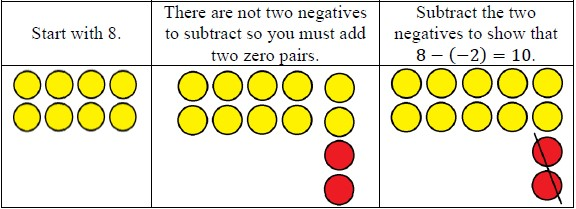• Instruction includes a graphic organizer to identify the key features. Based on the key features identified, ask students which form of an equation would be the best.
• For example, given the graph below, students can use an organizer to fill in some of the information.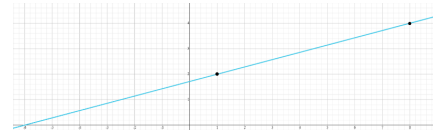• Once the information is filled in, students can write an equation of the line and determine the rest of the key features.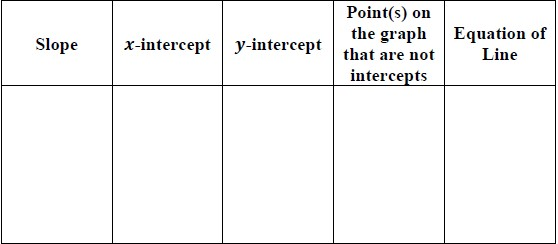Instructional Task 1 (MTR.2.1, MTR.3.1, MTR.5.1
• Jamie bought a car in 2005 for \$28,500. By 2008, the car was worth \$23,700.
• Part A. What function type could model the given situation?
• Part B. What is the rate of change in the vehicle’s worth per year?
• Part C. Create a model that describes this situation.

• Use the graph below to answer the following questions.• Part A. In order to write the equation that represents this line, what information do you need?
• Part B. Write a linear two-variable equation that represent the graph below. Justify why you choose the linear form to represent the graph.
• Part C. Write a real-world situation that could represent this graph.

### Instructional Items

Instructional Item 1
• Sharon is ordering tickets for an upcoming basketball game for herself and her friends. The ticket website shows the following table of ticket options.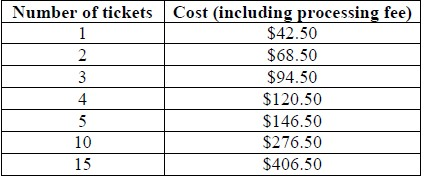• Write a linear two-variable equation to represent the total cost $C$ of $t$ tickets.

Instructional Item 2
• Write a linear two-variable equation that represents the graph below.*The strategies, tasks and items included in the B1G-M are examples and should not be considered comprehensive.

## Related Courses

This benchmark is part of these courses.
1200310: Algebra 1 (Specifically in versions: 2014 - 2015, 2015 - 2022, 2022 and beyond (current))
1200320: Algebra 1 Honors (Specifically in versions: 2014 - 2015, 2015 - 2022, 2022 and beyond (current))
1200370: Algebra 1-A (Specifically in versions: 2014 - 2015, 2015 - 2022, 2022 and beyond (current))
1200400: Foundational Skills in Mathematics 9-12 (Specifically in versions: 2014 - 2015, 2015 - 2022, 2022 and beyond (current))
7912080: Access Algebra 1A (Specifically in versions: 2014 - 2015, 2015 - 2018, 2018 - 2019, 2019 - 2022, 2022 and beyond (current))
1200315: Algebra 1 for Credit Recovery (Specifically in versions: 2014 - 2015, 2015 - 2022, 2022 and beyond (current))
1200375: Algebra 1-A for Credit Recovery (Specifically in versions: 2014 - 2015, 2015 - 2022, 2022 and beyond (current))
7912075: Access Algebra 1 (Specifically in versions: 2014 - 2015, 2015 - 2018, 2018 - 2019, 2019 - 2022, 2022 and beyond (current))

## Related Access Points

Alternate version of this benchmark for students with significant cognitive disabilities.
MA.912.AR.2.AP.2: Select a linear two-variable equation to represent relationships between quantities from a graph, a written description or a table of values within a mathematical or real-world context.

## Related Resources

Vetted resources educators can use to teach the concepts and skills in this benchmark.

## Formative Assessments

What Is the Point?:

Students are asked to explain the relationship between a given linear equation and both a point on its graph and a point not on its graph.

Type: Formative Assessment

Finding Solutions:

Students are asked to explain the relationship between a given linear equation and both a point on its graph and a point not on its graph.

Type: Formative Assessment

Functions From Graphs:

Students are asked to write a function given its graph.

Type: Formative Assessment

Writing a Function From Ordered Pairs:

Students are given a table of values and are asked to write a linear function.

Type: Formative Assessment

The Cost of Water:

Students are asked to write a function to model the relationship between two variables described in a real-world context.

Type: Formative Assessment

Tee It Up:

Students are asked to write an equation in three variables from a verbal description.

Note: This task may assess skills that exceed the general expectation for this mathematical concept at this grade level.  The task is intended for students who have demonstrated mastery within the scope of instruction who may be ready for a more rigorous extensions of the content. As with all materials, ensure to gauge the readiness of students or adapt according to students needs prior to administration.

Type: Formative Assessment

Tech Repairs:

Students are asked to write an equation in two variables from a verbal description.

Type: Formative Assessment

## Lesson Plans

Which Function?:

This activity has students apply their knowledge to distinguish between numerical data that can be modeled in linear or exponential forms. Students will create mathematical models (graph, equation) that represent the data and compare these models in terms of the information they show and their limitations. Students will use the models to compute additional information to predict future outcomes and make conjectures based on these predictions.

Type: Lesson Plan

Don't Blow the Budget!:

Students use systems of equations and inequalities to solve real world budgeting problems involving two variables.

Type: Lesson Plan

## Original Student Tutorials

Solving Systems of Linear Equations Part 6: Writing Systems from Context:

Learn how to create systems of linear equations to represent contextual situations in this interactive tutorial.

This part 6 in a 7-part series. Click below to explore the other tutorials in the series.

Type: Original Student Tutorial

Writing Equations in Two Variables:

Learn how to write equations in two variables in this interactive tutorial.

Type: Original Student Tutorial

Finding Solutions on a Graph:

Learn to determine the number of possible solutions for a linear equation with this interactive tutorial.

Type: Original Student Tutorial

## Perspectives Video: Professional/Enthusiast

Have a need for speed? Get out your spreadsheet! Race car drivers use algebraic formulas and spreadsheets to optimize car performance.

Type: Perspectives Video: Professional/Enthusiast

## Tutorials

Systems of Equations Word Problems Example 1:

This video demonstrates solving a word problem by creating a system of linear equations that represents the situation and solving them using elimination.

Type: Tutorial

Introduction to the Coordinate Plane:

In this video, you will learn about Rene Descartes, and how he bridged the gap between algebra and geometry.

Type: Tutorial

## Video/Audio/Animations

Using Systems of Equations Versus One Equation:

When should a system of equations with multiple variables be used to solve an Algebra problem, instead of using a single equation with a single variable?

Type: Video/Audio/Animation

Point-Slope Form:

The point-slope form of the equation for a line can describe any non-vertical line in the Cartesian plane, given the slope and the coordinates of a single point which lies on the line.

Type: Video/Audio/Animation

Two Point Form:

The two point form of the equation for a line can describe any non-vertical line in the Cartesian plane, given the coordinates of two points which lie on the line.

Type: Video/Audio/Animation

## MFAS Formative Assessments

Finding Solutions:

Students are asked to explain the relationship between a given linear equation and both a point on its graph and a point not on its graph.

Functions From Graphs:

Students are asked to write a function given its graph.

Tech Repairs:

Students are asked to write an equation in two variables from a verbal description.

Tee It Up:

Students are asked to write an equation in three variables from a verbal description.

Note: This task may assess skills that exceed the general expectation for this mathematical concept at this grade level.  The task is intended for students who have demonstrated mastery within the scope of instruction who may be ready for a more rigorous extensions of the content. As with all materials, ensure to gauge the readiness of students or adapt according to students needs prior to administration.

The Cost of Water:

Students are asked to write a function to model the relationship between two variables described in a real-world context.

What Is the Point?:

Students are asked to explain the relationship between a given linear equation and both a point on its graph and a point not on its graph.

Writing a Function From Ordered Pairs:

Students are given a table of values and are asked to write a linear function.

## Original Student Tutorials Mathematics - Grades 9-12

Finding Solutions on a Graph:

Learn to determine the number of possible solutions for a linear equation with this interactive tutorial.

Solving Systems of Linear Equations Part 6: Writing Systems from Context:

Learn how to create systems of linear equations to represent contextual situations in this interactive tutorial.

This part 6 in a 7-part series. Click below to explore the other tutorials in the series.

Writing Equations in Two Variables:

Learn how to write equations in two variables in this interactive tutorial.

## Student Resources

Vetted resources students can use to learn the concepts and skills in this benchmark.

## Original Student Tutorials

Solving Systems of Linear Equations Part 6: Writing Systems from Context:

Learn how to create systems of linear equations to represent contextual situations in this interactive tutorial.

This part 6 in a 7-part series. Click below to explore the other tutorials in the series.

Type: Original Student Tutorial

Writing Equations in Two Variables:

Learn how to write equations in two variables in this interactive tutorial.

Type: Original Student Tutorial

Finding Solutions on a Graph:

Learn to determine the number of possible solutions for a linear equation with this interactive tutorial.

Type: Original Student Tutorial

## Tutorials

Systems of Equations Word Problems Example 1:

This video demonstrates solving a word problem by creating a system of linear equations that represents the situation and solving them using elimination.

Type: Tutorial

Introduction to the Coordinate Plane:

In this video, you will learn about Rene Descartes, and how he bridged the gap between algebra and geometry.

Type: Tutorial

## Video/Audio/Animations

Using Systems of Equations Versus One Equation:

When should a system of equations with multiple variables be used to solve an Algebra problem, instead of using a single equation with a single variable?

Type: Video/Audio/Animation

Point-Slope Form:

The point-slope form of the equation for a line can describe any non-vertical line in the Cartesian plane, given the slope and the coordinates of a single point which lies on the line.

Type: Video/Audio/Animation

Two Point Form:

The two point form of the equation for a line can describe any non-vertical line in the Cartesian plane, given the coordinates of two points which lie on the line.

Type: Video/Audio/Animation

## Parent Resources

Vetted resources caregivers can use to help students learn the concepts and skills in this benchmark.